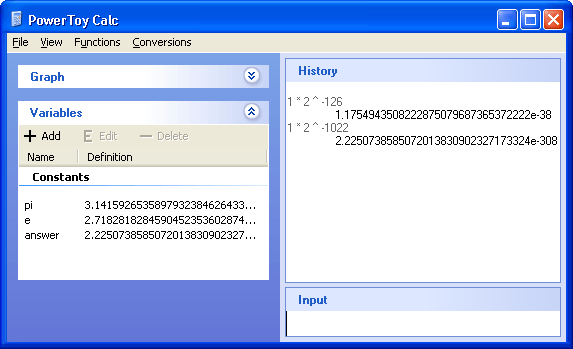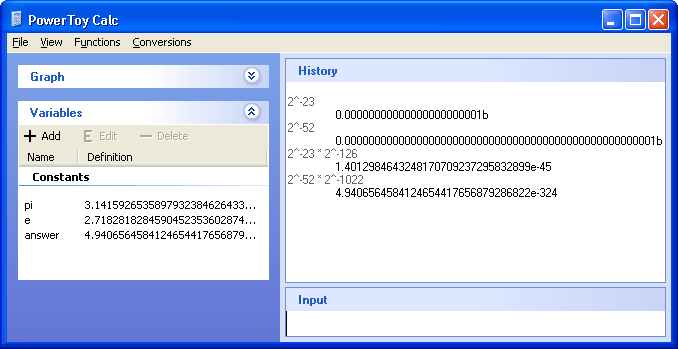Smallest Positive Floating Point Values

Wednesday, July 26, 2006
By: Jason Doucette

What are the limitations of the floating point data types in C and C++?  C and C++ typically is use IEEE 32-bit single-precision for float, and IEEE 64-bit double-precision for double.  If you take a look inside of FLOAT.H, you can see the smallest and largest possible positive values for float and double:

#define FLT_MIN         1.175494351e-38F        /* min positive value */
#define FLT_MAX         3.402823466e+38F        /* max value */
#define DBL_MIN         2.2250738585072014e-308 /* min positive value */
#define DBL_MAX         1.7976931348623158e+308 /* max value */

You'll notice for both float and double that the exponent seems to go as high into the positives as it does into the negatives.  Let's try inverse one and seeing if it matches the other:

#include <stdio.h>
#include <float.h>
int main()
{
double minimum = DBL_MIN;
printf("min = %.17g\n",minimum);

double maximum = DBL_MAX;
printf("max = %.17g\n",maximum);

double inverse_max = 1.0 / maximum;
printf("1.0/max = %.17g\n",inverse_max);
}

The output is as follows:

min = 2.2250738585072014e-308
max = 1.7976931348623157e+308
1.0/max = 5.5626846462680035e-309

Whoa!  The inverse of the maximum float value is smaller than the minimum positive value!  How could that happen?

Possible Causes

The FPU uses 80-bit floating point values internally for intermediate calculations.  It can store numbers in the range 3.36210314311209350626e-4932 to 1.18973149535723176509e+4932.  So, the FPU can easily store the value 5.5626846462680035e-309 internally.  So, perhaps it is passing this 80-bit value to printf().  printf() is a variable argument list function, which can accept values of any type, so this is possible, right?  No, because variable argument list functions can only accept double values; this is enforced by the compiler.  The value passed to printf() really is a double value.  (You can prove this by storing it temporarily into a double variable, or by wrapping printf() with your own function that accepts a double type.  Ensure you compile in DEBUG mode with no optimizations to ensure inlining does not occur.)

How can the define and comment within FLOAT.H — a file that millions of programmers use — be wrong?

Technical Details

Let's determine, by hand, what the smallest floating point values should be.  Floating point numbers are computed like so:

value = sign * mantissa * 2 ^ exponent

The sign = -1 or +1.  We are dealing only with the limitations of the magnitude, so let's assume this is +1 in all cases.  The same results will be true for negative numbers.  The exponent = -126..127 for 32-bit float, and  -1022..1023 for 64-bit double.  The mantissa (also known as the significand) is >= 1.0 and < 2.0.  Thus the smallest possible positive float and double are such that the exponent and mantissa contain their smallest values:

smallest float = 1 * 2 ^ -126 = 1.175494351e-38
smallest double =  1 * 2 ^ -1022 = 2.2250738585072014e-308This matches the defines in FLOAT.H.  So, how can we store something smaller than these values?

Because, there are two methods of storting a floating point value.

The most common method is stored as a normalized number.  It is stored such that the mantissa is always >= 1.0, and less then 2.0.  The mantissa is 'normalized'.  Because, in binary, this implies the mantissa always starts with a 1 bit (since 1.0 in binary = 1.000..., and 1.999... in binary = 1.111...), we need not store this 1.  It is implied.  Thus this format is a 'packed' format.

The less common method is stored as a denormalized number.  As you probably guessed, the mantissa is not normalized.  It is not within the range 1.0 <= mantissa < 2.0.  Thus, its first bit is not necessarily a 1.  Thus, it must be stored.  Therefore, this is an 'unpacked' format.  How do we know when a number is stored in this format?  When the exponent is stored internally as all 0's, it signifies that this is a special case, and the number is denormalized.  (The exponent still represents the value -126 for float, and -1022 for double, however).  The other special case is when the exponent is stored as all 1's internally, which signifies infinity or NaN (Not a Number).  This is why the exponent ranges are from -126..127, which is only 254 values instead of 256, (and -1022..1023, which is only 2046 values instead of 2048), because 2 values are 'special'.

The denormalized number method is required to store zero.  If the mantissa was always: 1.0 <= mantissa < 2.0, then there is no way zero can be stored.  With a denormalized format, the mantissa can = 0, thus the equation "value = sign * mantissa * 2 ^ exponent" can result in 0.

However, as a side effect of the denormalized number, the mantissa can be a numerous amount of values from 0 to just under 1.0.  Let's look at the smallest non-zero value for the mantissa.  This occurs when all the mantissa bits are 0 except for the least significant bit.  float stores its mantissa in 23 bits.  double stores its mantissa in 52 bits.  The decimal place within these bits occur immediately before the first bit.  Thus (note that the "b" postfix signifies binary, as opposed to decimal):

smallest denormalized float mantissa = .00000000000000000000001b = 2^-23
smallest denormalized double mantissa = .0000000000000000000000000000000000000000000000000001b = 2^-52

Thus, the smallest possible denormalized float and double values are:

smallest denormalized float = 2^-23 * 2^-126 = 1.401298464e-45
smallest denormalized double = 2^-52 * 2 ^ -1022 = 4.9406564584124654e-324Note that the key issue here is that the  that the precision dwindles as the denormalized numbers get smaller, since you are using less and less bits to store the precision of the magnitude of the number, and you are using more and more bits to store leading 0's.